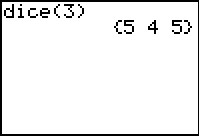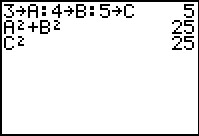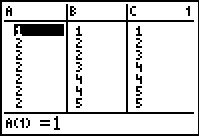# Activities

••• ##### Subject Area

• Math: Middle Grades Math: Algebraic Thinking

• ##### Author6-8

45 Minutes

• ##### Device
• TI-73 Explorer™
• TI-Navigator™

TI Connect™

## Is It A Triangle?#### Activity Overview

In this activity, students will investigate properties of triangles, classify the various types of triangles, and determine if specific side lengths will make a triangle or not.

#### Key Steps

•Students will investigate properties of triangles by randomly generating possible side lengths for triangles. They will experimentally try to make triangles with the randomly generated numbers and then make conjectures about triangle inequalities.

•After creating triangles and discussing the possible sides that create triangles, students will then classify triangles based on their characteristics (side lengths and angles).

•Students will then combine all their data and include any possible missing sets to make an organized list with all possible triangles with side lengths between 1 and 6. They will use the calculator list to enter all 28 triangles and test to make sure they are triangles using the triangle inequality rule discovered.